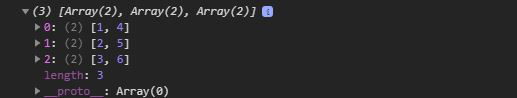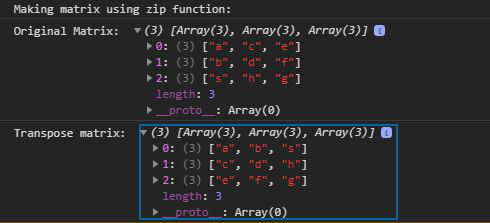Related Articles

# D3.js transpose() Function

• Last Updated : 19 Jul, 2020

The transpose() function in D3.js is used to return the transpose of a 2D array. This function helps to visualize the data and making graphs and charts.

Syntax:

Hey geek! The constant emerging technologies in the world of web development always keeps the excitement for this subject through the roof. But before you tackle the big projects, we suggest you start by learning the basics. Kickstart your web development journey by learning JS concepts with our JavaScript Course. Now at it's lowest price ever!

```d3.transpose(Matrix);
```

Parameters: This function accept a single parameter as mentioned above and described below:

• Matrix: This parameter holds a matrix as a 2d array

Return value: It returns an array or the matrix.

Below given are a few examples of transpose function.

Example 1: Without using zip function to form a matrix.

## HTML

 ```<``html` `lang``=``"en"``>``<``head``>``  ``<``meta` `charset``=``"UTF-8"``>``  ``<``meta` `name``=``"viewport"` `        ``content``=``"width=device-width, initial-scale=1.0"``>``  ``<``title``>D3.js transpose() Function````<``body``>``  ````  ``<``script` `type` `= ``"text/javascript"` `          ``src` `= ``"https://d3js.org/d3.v4.min.js"``>``  ````  ``<``script``>``    ``console.log(d3.transpose([[1,2,3],[4,5,6]]))``  ```````

Output:Example 2: Using zip function to form a matrix.

## HTML

 ```<``html` `lang``=``"en"``>``<``head``>``  ``<``meta` `charset``=``"UTF-8"``>``  ``<``meta` `name``=``"viewport"` `        ``content``=``"width=device-width, initial-scale=1.0"``>``  ``<``title``>D3 transpose() Function````<``body``>``   ` `  ````  ``<``script` `type` `= ``"text/javascript"` `          ``src` `= ``"https://d3js.org/d3.v4.min.js"``>``  ````  ``<``script``>``    ``console.log("Making matrix using zip function: ")``    ``let m=d3.zip(["a","b","s"],["c","d","h"],["e","f","g"]);``    ``console.log("Original Matrix: ",m)``    ``console.log("Transpose matrix: ",d3.transpose(m))``  ```````

Output:Supported Browsers: